# 1.11 Signal Sources

There are a variety of test equipment that can create signals with ranges of different characteristics.  Three classes of these signal sources we will talk about today: Signal Generators, Pulse Generators, and function Generators.

Signal Generators produce sine waves while allowing you to define the amplitude, frequency, and even allowing for these to vary overtime.Pulse Generators create pulses, allowing for you to vary the amplitude, pulse width, frequency, and even rise time and fall time.Function Generators allow for you to create more complex waves, in a variety of shapes: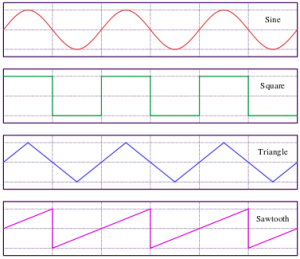# 1.09 Other signals and 1.10 Logic Levels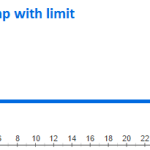Let’s cover two sections today:
1.09 Other signals
1.10 Logic Levels

We have been looking at Voltage as a function of time and seeing how it will look on a graph.  The past few days we have talked about graphs that are sine wave.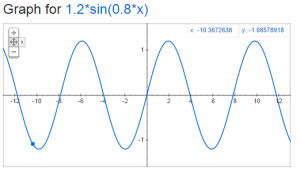But there could be other ways that this could look too.  Consider if voltage started out at zero and continued to increase.  It would look something like this:But it is not possible to increase voltage indefinitely and so the graph would really look like this:  (Ramp with limit)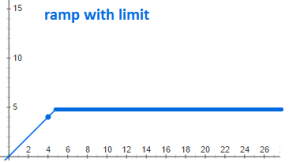But there are other interesting graphs to.  Consider the case of voltage increasing to a point, and then when it reaches a certain point it goes to zero and then begins again.  Let’s say you have a circuit that triggers at a certain voltage, and a capacitor that you are charging up till that point.  It might looks something like this sawtooth wave: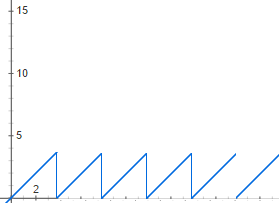But you can also imagine a case where it reaches a point and decreases at the same linear rate.  This would look like a triangle wave: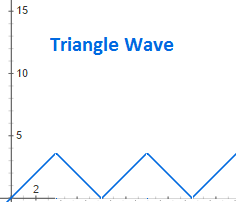And then there is noise, a complex, background static that we always need to consider and usually minimize:Square waves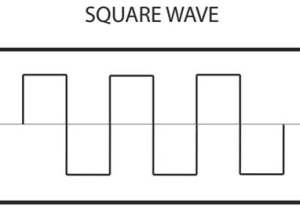StepsSpikesBut in digital electronics we concern ourselves with logic levels and interrupting a particular voltage as either a true or a false; as a on or a off; as a 1 or 0.  Most digital electronics have a threshold voltage.  Above that voltage it is considered to be true/on/1.  Below that voltage it is considered to be false/off/0.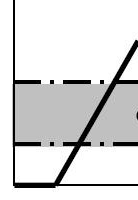# 1.08 Signal amplitudes and decibels

Peak-to-peak amplitude (pp amplitude) is twice the amplitude. This is the measurement of the amplitude from the bottom peak to the top peak as illustrated below:root-mean-square amplitude (rms amplitude) has different formulas based upon the shape of the signal.  For sine waves only it is given by:Decibels is a comparison between two signals.

When comparing amplitude use this formula:When comparing the power use this formula:Sometimes decibels are presented as units.  This is because their is an implicit signal that is being used for comparison.  Here are some common ones:

a) dBV:  1 volt rms

b) dBm – the voltage corresponding to 1mW with an assumed load impedance

c) small noise generated by a resistor at room temperature

# 1.07 Sinusoidal signals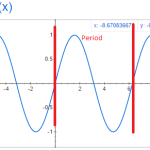The next few sections in The Arts of Electronics deals with signals.  What are the characteristics of signals?  Amplitude, frequency, shape, etc.

The values we are looking at is voltage as a function of time.  The most basic signal is the sinusoidal signal.  Have you ever graphed y=sin(x) and end up with: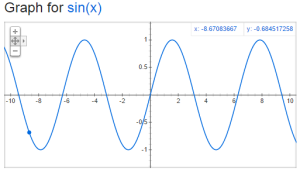But how would you compare this wave to the following: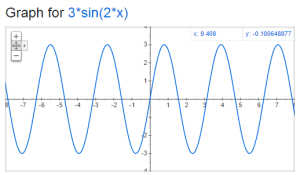There are two key things to think about when comparing sine waves:  frequency and amplitude.    The amplitude is the distance from y=0 to the top of the sine wave.  The frequency is how many times the wave repeats in a given period of time.  We typically use the unit hertz (Hz) for frequency, which is one cycle (or period) per second.  Lets take a look at period and amplitude in our original sine graph:The formula that you should remember is

V=A*sin(2*pi*f*t)

A is the amplitude in volts, f is the frequency in Hz and t is the time in seconds.

# 1.06 Small-signal resistanceOhm’s law is R=V/I.  For the ordinary resistance, this is a constant proportion for a large range of V.  But this is not true for all devices.  Today we look at the Zener diode which has a high resistance up to a point and then almost no resistance for a large range of V.  Also, we look at the tunnel diode (also known as the Esaki diode) which actually has a negative resistance over a particular range of V.

# 1.05 Thevenin’s equivalent circuit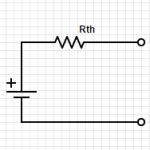Any two terminal network of voltage sources and resistors can be replace by a single voltage source in combination with a single resistor.  This is called the Thevenin’s equivalent circuit: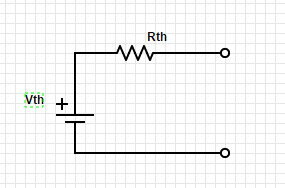The first step is to identify the two terminals of the network.

Then to figure out Rth, replace any voltage sources with short circuits and any current sources with open circuits.

Calculate the voltage between the two terminals.

Then figure out Vth between the two terminals.

To figure this out using testing and measuring, it is only necessary to find the voltage at the two terminals with power on, and the resistance with power off.

This can greatly simplify circuit analysis of complex circuits.

Check out videos on YouTube with more detailed information:

# 1.04 Voltage and current sourcesLet’s take a look at the symbols for voltage sources and current sources. Also, let’s discuss the properties of the ideal voltage source and the ideal current source.

The ideal voltage source will maintain the same voltage between its terminals regardless of the load. This is easiest with an open circuit but becomes impossible with a short circuit.

The ideal current source will maintain the same current regardless of the resistance of the load. This is easy with an open circuit but becomes more and more difficult as the resistance increases.

# 1.03 Voltage Dividers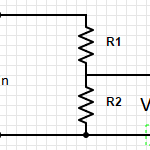Voltage Dividers take an input voltage and gives you a smaller, fractional output voltage. This can be useful for volume control or when interfacing between logic circuits that run at 5V with new/lower power devices that run at 3.3V.

A voltage divider is a simple circuit made up of only two resistors (R1 & R2 in the diagram below).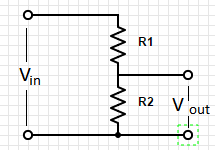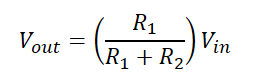# 1.02 Relationship between voltage and current: resistorsArt of Electronics 2nd Edition p 4-7

What you MUST learn

Resistance (represented in formulas as “R”) measured in Ohms which is represented using the symbol: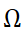Resistance is the relationship between Current (I) and Voltage (R).

This relationship is defined by Ohm’s law:  R = V/I.

Circuit diagrams represent resistors as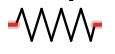Resistors in Series: (Always bigger)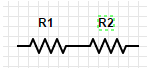R= R1 + R2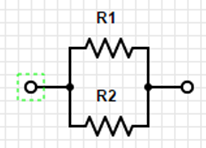Resistors in Parallel: (Always smaller)

R = (R1*R2)/(R1+R2)

Shortcut #1:

A large resistor in series with a small resistor has the resistance of the larger one, roughly.

A large resistor in parallel with a  small resistor has the resistance of the smaller one, roughly.

Try to develop an intuitive sense of the voltage and current in the parts of the circuit.  Do not try to calculate to be extremely precise because the precision of the components will make this pointless.  Also, good circuit design should be tolerant of variations.

Power:

P=IV

Using Ohm’s law you get:

P=(I^2)R

P=(V^2)/R

Things good to learn

The inverse of resistance is conductance (represented by “G” in formulas)  G=1/R

Restatement of Ohm’s law:  I=GV

Conductance is measured in siemenssiemens is also known as mho (ohm spelled backwards)

# 1.01 Voltage and Current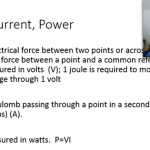Art of Electronics 2nd edition, pages 2-4

Overview

Voltage & Current describe two aspects of electronic circuits that are fundamental to understand and on which everything else will build on.

What you MUST learn

Voltage refers to a force that when applied across a circuit causes current to flow.  Voltage is measured in volts (using the symbol V) also called a “potential difference”.  It is measured across two points in a circuit.  A joule of work is needed to move a coulomb of charge through a potential difference of one volt. A coulomb is the charge of about 6×10^18 electrons.

Current (symbol: I) is the measure of electronics through a point in a circuit.  It is measured in amperes (amps) (symbol: A).  1 ampere (amp) is 1 coulomb of charge passing through a point per second.

Conservation of Charge:  Kirchhoff’s current law

The sum of currents into a point or node is equal to the sum of the currents out of a point or node.  This is referred to as Kirchhoff’s current law.

Kirchhoff’s voltage law

The sum of voltage drops through one branch of a circuit will equal the sum of voltage drops across the other branches of the circuit.

P=VI

Power (work per unit time), measured in watts.  (1 W = 1 J/s)

P=VI = (work/charge) x (charge/time) = work/time

P=VI= (1 joules/1 coulomb) x (1 coulomb/1 second) = 1 joules/second = watt.

Things good to learn

Voltage is usually written with the symbol V but sometimes E is used.   Voltage is also called a “potential difference” or electromotive force (EMF).

Prefixes:

 Multiple Prefix Symbol 10^12 tera T 10^9 giga G 10^6 mega M 10^3 kilo k 10^(-3) milli m 10^(-6) micro µ 10^(-9) nano n 10^(-12) pico p 10^(-15) femto f

When abbreviating a unit with a prefix, the unit follows the multiplier with no space and the unit is capitalized.  However, no capitalization for both prefix and unit when spelled out.  1mW = 1 milliwatt

1 MV = 1 megavolt

Related information and insights from my other books

Introductory Circuit Analysis 9th edition – chapter 2

Structure of the atom:

nucleus is made up of protons (that have a positive charge) and neutrons that have a negative charge.  The proton and neutron are relatively the same mass.  But the electron is considerably smaller (1836 times smaller) than the proton but has a negative charge that is equal to the positive charge of the proton.

Other things of interest

If you need more help

Voltage

Current

Joule

If you wanted to know

Who defined:

Voltage

Volt

Current

amp

joule

watt

multiplier prefixes

People who contributed:

Watt